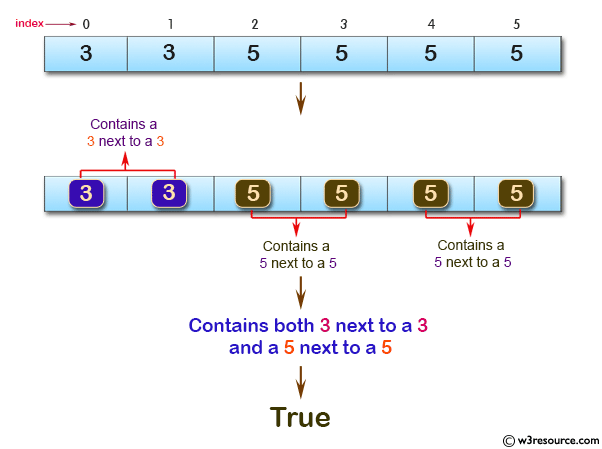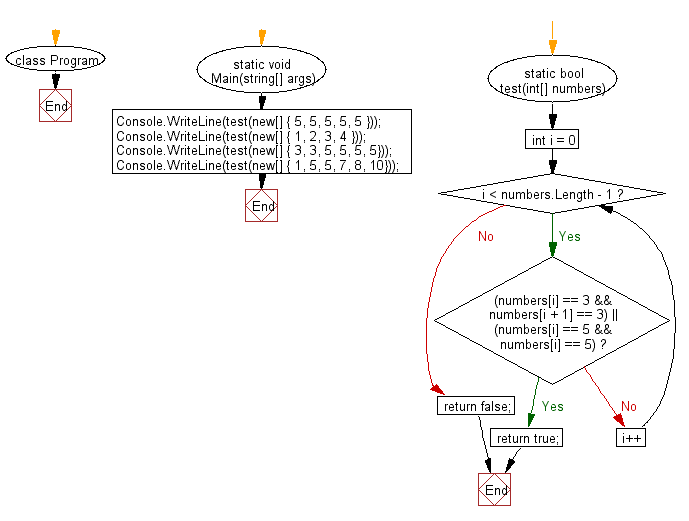﻿ C# - If an array of contains a 3 or a 5 next to each other# C# Sharp Basic Algorithm Exercises: Check whether an array of integers contains a 3 next to a 3 or a 5 next to a 5 or both

## C# Sharp Basic Algorithm: Exercise-119 with Solution

Write a C# Sharp program to check whether an array of integers contains a 3 next to a 3 or a 5 next to a 5 or both.

Pictorial Presentation:Sample Solution:

C# Sharp Code:

``````using System;
namespace exercises
{
class Program
{
static void Main(string[] args)
{
Console.WriteLine(test(new[] { 5, 5, 5, 5, 5 }));
Console.WriteLine(test(new[] { 1, 2, 3, 4 }));
Console.WriteLine(test(new[] { 3, 3, 5, 5, 5, 5}));
Console.WriteLine(test(new[] { 1, 5, 5, 7, 8, 10}));
}
static bool test(int[] numbers)
{
for (int i = 0; i < numbers.Length - 1; i++) if ((numbers[i] == 3 && numbers[i + 1] == 3) || (numbers[i] == 5 && numbers[i] == 5)) return true;
return false;
}
}
}
```
```

Sample Output:

```True
False
True
True```

Flowchart:C# Sharp Code Editor:

Improve this sample solution and post your code through Disqus

What is the difficulty level of this exercise?

Test your Programming skills with w3resource's quiz.

﻿